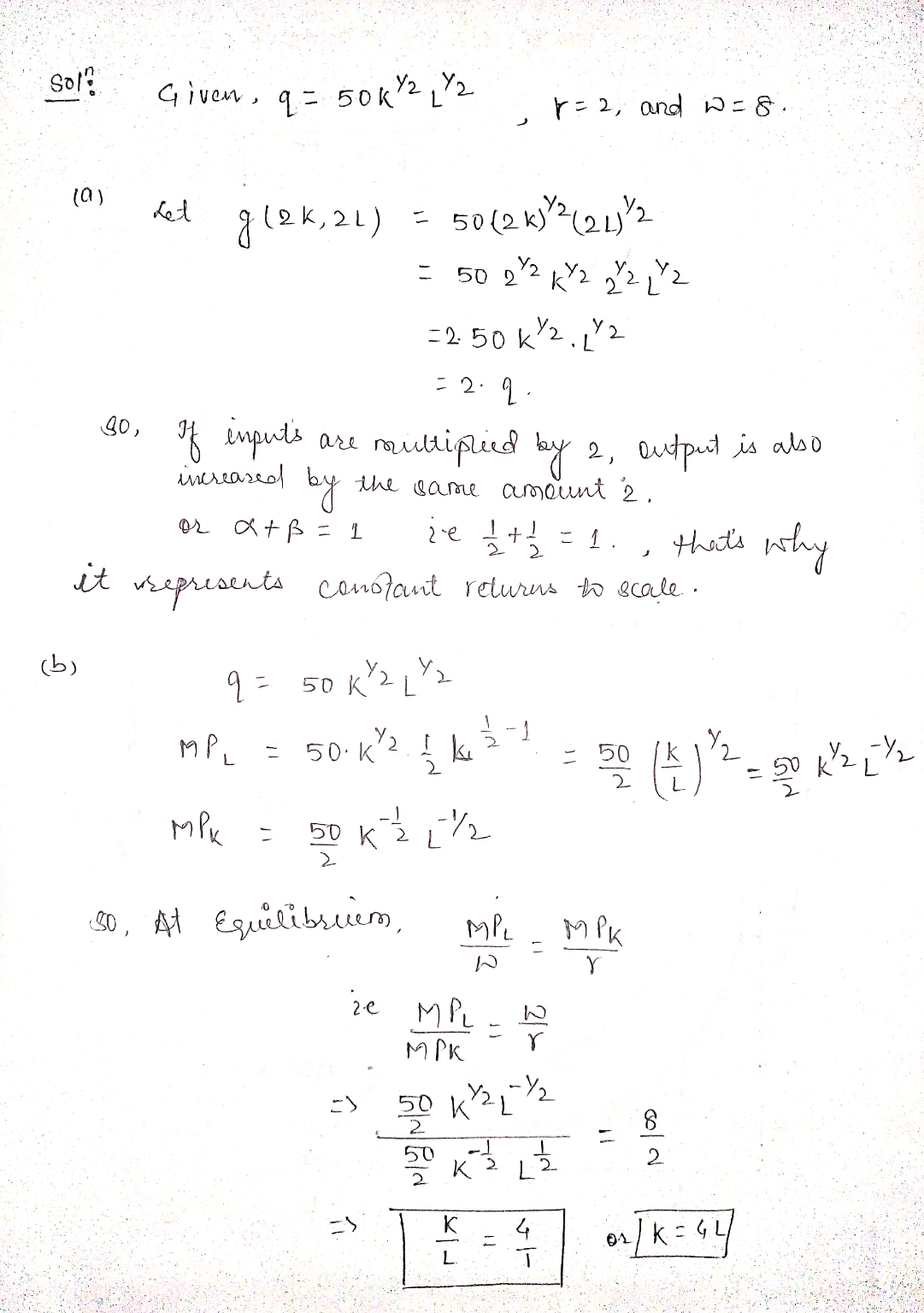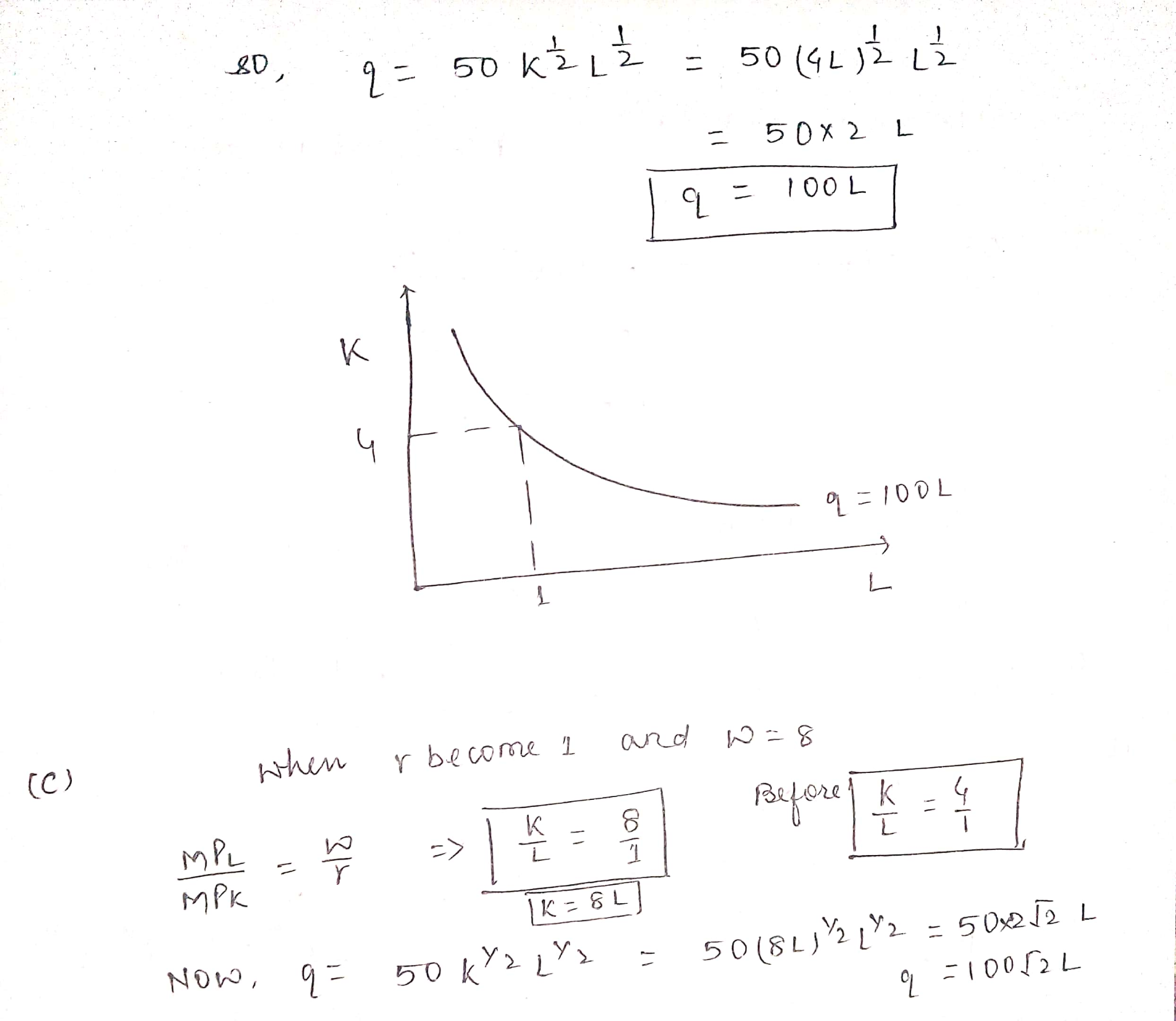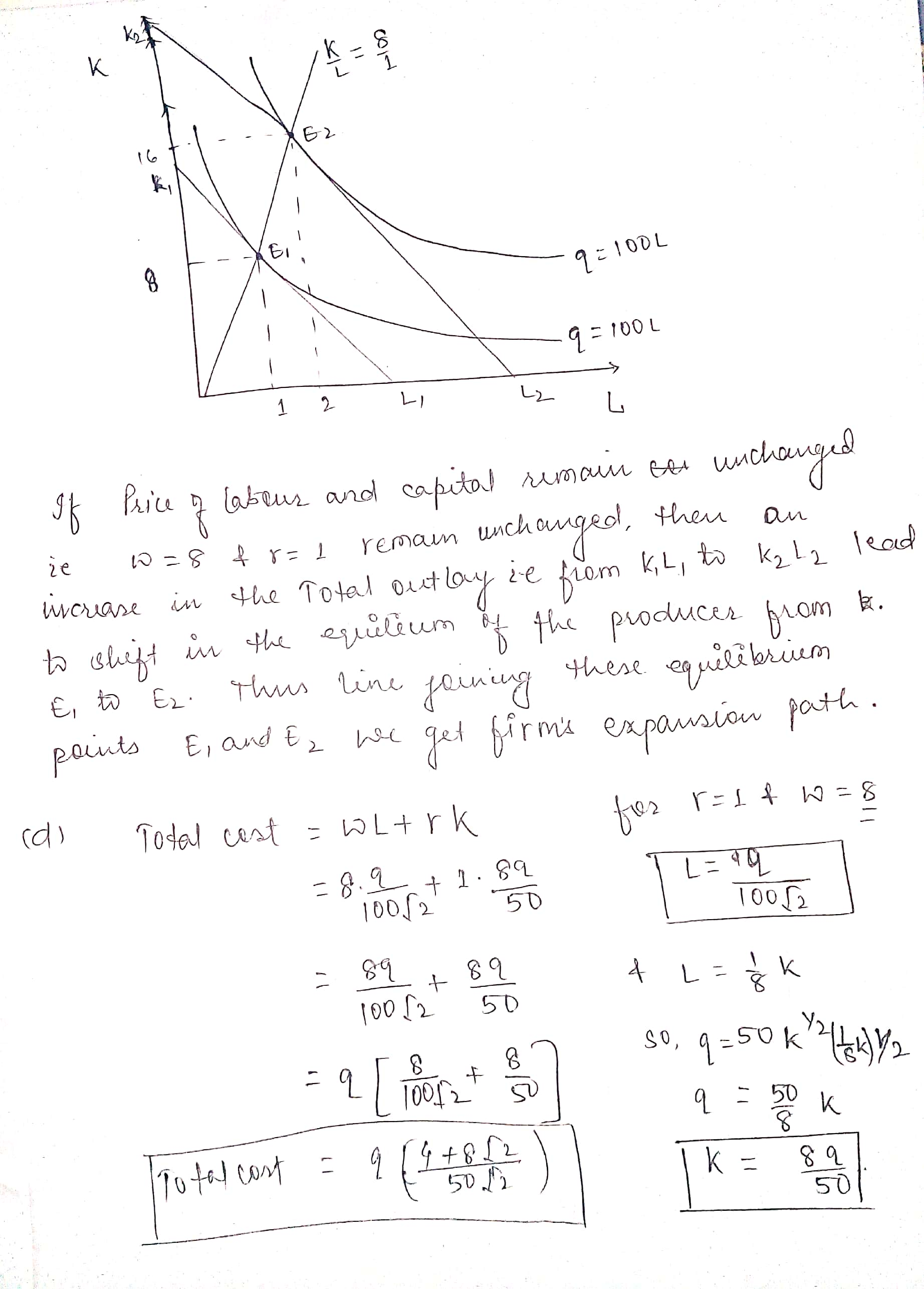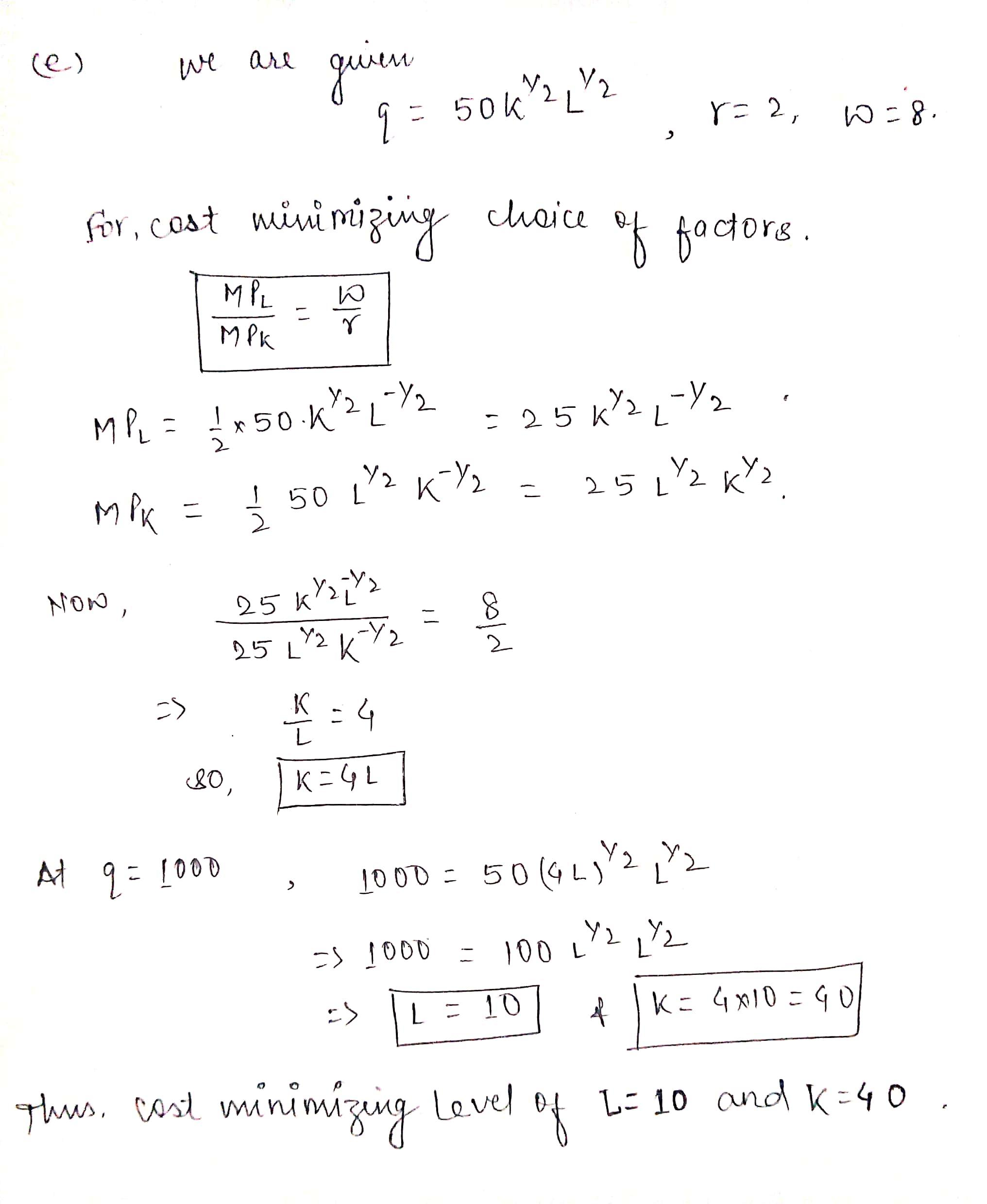In: Economics

# Suppose your firm uses 2 inputs to produce its output: K (capital) and L (labor). the...

Suppose your firm uses 2 inputs to produce its output: K (capital) and L (labor). the production function is q = 50K^(1/2)L^(1/2). prices of capital and labor are given as r = 2 and w = 8

a) does the production function display increasing, constant, or decreasing returns to scale? how do you know and what does this mean?

b) draw the isoquants for your firms production function using L for the x axis and K for y. how are the factors K and L?

c) derive the expansion path equation. represent it graphically. how does the expansion path change when r = 1 and w = 8?

d) find the total cost function as a function of quantity

e) represent the firms cost minimizing choice of factors to produce a given quantity q in a diagram. if q = 1000, calculate K and L

## Solutions

##### Expert Solution## Related Solutions

##### There is a firm who manufacturers and uses capital (K) and labor (L) to product output...
There is a firm who manufacturers and uses capital (K) and labor (L) to product output Q such that Q=10KL. The unit price for K and L are w = $15 and r =$5, respectively. 1).Does the firm’s production exhibit decreasing, constant, or increasing returns to scale? 2)What is the optimal input bundle (K*, L*) to produce 480 unit of output? 3)Derive the long run cost function.
##### A competitive firm uses two inputs, capital (?) and labour (?), to produce one output, (?)....
A competitive firm uses two inputs, capital (?) and labour (?), to produce one output, (?). The price of capital, ??, is $1 per unit and the price of labor, ?? , is$1 per unit. The firm operates in competitive markets for outputs and inputs, so takes the prices as given. The production function is ?(?, ?) = 3? 0.25? 0.25. The maximum amount of output produced for a given amount of inputs is ? = ?(?, ?) units....
##### A firm produces output using capital (K) and labor (L). Capital and labor are perfect complements...
A firm produces output using capital (K) and labor (L). Capital and labor are perfect complements and 1 unit of capital is used with 2 units of labor to produce 1 unit of output. Draw an example of an isoquant. If wages and rent are $2 and$3, respectively, what is the Average Total Cost? A firm has a production function given by Q=4KL where K, L and Q denote capital, labor, and output, respectively. The firm wants to produce...
##### A firm uses two inputs, labor and capital, to produce a good. To keep up with...
A firm uses two inputs, labor and capital, to produce a good. To keep up with the story, let zℓ ≥ 0 denote the units of labor and zk ≥ 0 the units of capital. The firm’s technology is expressed as a production function f(zℓ, zk) = 20 z1/5 ℓ z3/5 k . Let w > 0 and r > 0 be the cost of hiring a unit of labor and a unit of capital, respectively. (a) Find the technical...
##### . A firm uses the inputs of fertilizer (sacks), labor and hothouses to produce roses. Suppose...
. A firm uses the inputs of fertilizer (sacks), labor and hothouses to produce roses. Suppose that when the quantity of labor and hothouses is fixed, the relationship between the quantity of fertilizer (F) used and the number of roses (TP) produced is given by the following table.         F      TP           APF         MPF         0        0         10     1,100         20     2,200         30     4,800         40     7,600         50     9,800         60   11,600         70   12,200         80   11,800...
##### Consider an economy that uses two factors of production, capital (K) and labor (L), to produce...
Consider an economy that uses two factors of production, capital (K) and labor (L), to produce two goods, good X and good Y. In the good X sector, the production function is X = 4KX0.5 + 6LX0.5, so that in this sector the marginal productivity of capital is MPKX = 2KX-0.5 and the marginal productivity of labor is MPLX = 3LX-0.5. In the good Y sector, the production function is Y = 2KY0.5 + 4LY0.5, so that in this sector...
##### A firm discovers that when it uses K units of capital and L units of labor...
A firm discovers that when it uses K units of capital and L units of labor it is able to       produce q=4K^1/4 L^3/4 units of output. Continue to assume that capital and labor can be hired at $40 per unit for labor and$10 for capital. In the long run if the firm produces 600 units of output, how much labor and capital will be used and what is the LR Total cost of production?
##### A firm discovers that when it uses K units of capital and L units of labor...
A firm discovers that when it uses K units of capital and L units of labor it is able to       produce q=4K^1/4 L^3/4 units of output. a) Calculate the MPL, MPK and MRTS b) Does the production function (q=4K^1/4 L^3/4) exhibit constant, increasing or decreasing returns to scale and why? c) Suppose that capital costs $10 per unit and labor can each be hired at$40 per unit and the firm uses 225 units of capital in the short run....
##### A firm produces output y using two factors of production (inputs), labour L and capital K....
A firm produces output y using two factors of production (inputs), labour L and capital K. The firm’s production function is ?(?,?)=√?+√?=?12+?12. The wage rate w = 6 and the rental price of capital r = 2 are taken as parameters (fixed) by the firm. a. Show whether this firm’s technology exhibits decreasing, constant, or increasing returns to scale. b. Solve the firm’s long run cost minimization problem (minimize long run costs subject to the output constraint) to derive this...
##### Suppose output, Q, is produced by labor, L, and capital, K, according to the following function:...
Suppose output, Q, is produced by labor, L, and capital, K, according to the following function: Q = K ½ L½.. Suppose the firm sells each unit of output in a competitive market for a price P = $100. Suppose the firm hires each unit of labor in a competitive market for a wage W =$25. Suppose the firm has to make do for now with a stock of capital K = 49; moreover, suppose each unit of capital...